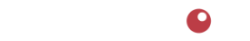# Demystifying Margin and Markup

Here's a little riddle: What two English words start with the letters "m-a-r", contain the same number of syllables and letters, and if they get confused can cost a business lots of money? Just in case the title of this article didn't tip you off to the answer, the words are "margin" and "markup".

We have found that many people get the concepts of margin and markup mixed up and often calculate their margin incorrectly. This confusion can lead to problems because if someone is basing important business decisions on margin but accidentally calculates markup, the outcome may seem more favorable than it actually is.

This is unfortunate for three reasons:
1. Having an understanding of the difference is essential for nearly anyone running a food business.
2. Calculating margin incorrectly generally leads to lower profits because product pricing strategies are often designed to achieve a target margin.
3. It doesn't need to be that way! The math to calculate margin is very straightforward and easy to do.

Given how similar both words are, it's easy to see how they can be confused especially considering both are related to cost, price, and profit. Spoiler alert: by the end of this article we hope we will have convinced you to focus only on margin and never, ever speak of markup again!

Margin vs Markup

To get clear on the concepts of margin and markup, we'll provide a brief explanation of each:

Technically, margin is simply the difference between the amount it costs to make (or purchase) a product and the price it sells for. We say "technically" because when most business people say "margin", they are actually talking about "margin percent". Margin percent is the percentage of the selling price that is profit.

Markup is used when a firm buys goods from a supplier at one price and marks up the price to sell to customers. Markup was a common approach for merchandising operations in the "old days" but nowadays, most businesses include margin in their decision-making - not markup.

Margin is the percentage of the selling price that is profit. If one thinks they're calculating margin but instead calculates markup, they will think their margin is higher than it actually is.

In our experience the overwhelming majority of food retailers, distributors, wholesalers, and suppliers talk in terms of margin. And it's worth repeating that when someone says "margin" they usually are referring to "margin percent". Markup, on the otherhand, is rarely used in food businesses.

Another advantage of getting in the habit of calculating percent margin is that it invites comparisons of products at different price points and allows you to determine their relative profitability.

The Math of Margin

First determine profit.
If your product costs \$2 per unit to make and the selling price \$3 per unit, the profit margin is \$1.
Price - Cost = Profit
3.00 - 2.00 = 1.00

In the above example, our profit is \$1.00.
You may notice that we refer to cost as the amount we pay for something and price as the amount we sell it for. For food product manufacturers, cost is the amount it costs to make one unit of product.

Next determine margin.
To calculate the margin percent, simply divide profit by selling price.
Profit / Price = Margin
1.00 / 3.00 = 0.3333

In the above example, our margin is 33.33%.

#### Margin Calculation: The Complete Formula

Margin % = (Price - Cost) / Price

Remember: calculate profit first (Price - Cost) then divide profit by Price to get Margin %.

Now that you possess the profound knowledge of how to calculate margin, we'll reiterate its definition:
Margin is the percentage of the selling price that is profit.

Divide by Price Not Cost

Notice that to calculate margin, we divided profit by price, not by cost. If we divided profit by cost we would be calculating markup and that's not what we want. Earlier we stated that confusing margin and markup could cause trouble. That's because if one thinks they're calculating margin but instead calculates markup, they'll likewise think their margin is higher than it actually is. In the above example, if we had incorrectly divided profit by cost (1.00 / 2.00 = 0.50), we would come away thinking our margin is 50% when it is actually only 33.33%.

Worse, if you set prices with a target margin in mind but you mistakenly calculate markup instead, your prices will be lower than they should be to achieve the target margin. For example, if we're targeting a 33.3% margin but calculate it by dividing profit by cost instead, we would set our unit price per unit at 2.66. This would lead us to believe (and possibly tell investors) that our margin is 33.3% instead of the actual margin of 24.81%. The difference can be significant.

Invent a Silly Rhyme

To keep the calculation straight in your mind, you might invent a rhyme so you can remember it later (the sillier it is, the easier it is to remember). Something like "Divide profit by price for a margin that's nice. Divide profit by cost and money will be lost."

Because nearly everyone in the food business uses margin instead of markup, Food Methods advises its clients to do the same. The business decisions you make about pricing and profits should nearly always involve margins and not markup.

Last Updated: 04/24/20204110 SE Hawthorne Blvd. #739

Portland OR 97214

503.234.7199

Notice | Privacy